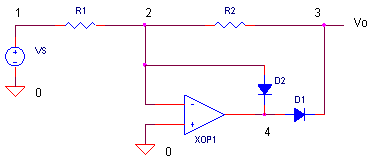eCircuit  Center

Op Amp Precision Half-wave Rectifier

CIRCUITRectifiers are often called into action to measure signal strength. Rectify an AC signal, pass it through a low-pass filter and the resulting DC level represents some measure of the signal's magnitude. Although the series diode is the classic rectifier, it can't rectify signals smaller that it own forward voltage! But what if your expected amplitude can be as low as 100 mV? Op amps to the rescue! The advantage of op amp circuits lies in their ability to compensate for non-linear devices in the feedback loop. Combining the rectifying action of a diode with the accuracy of an op amp, this circuit creates a precision rectifier.

INVERTING HALF-WAVE RECTIFIER

During the negative half-cycle of a sinewave input, the output should be positive. During the positive half-cycle, the output should be zero. The circuit that accomplishes this amazing feat looks like the inverting amplifier with a couple of diodes added. Why two? Only one actually does the rectifying action. The other simply keeps the op amp in control while the signal output holds at zero.

For the negative half-cycle input, the op amp output goes positive forcing D1 to turn ON and D2 to shut OFF.It looks and acts just like the inverting amplifier, except for a diode in a series with the op amp's output pin. But no need to worry, the output pin adjusts itself higher (by the diode's forward voltage, about 0.6V ) to get the right voltage at the Vo. The classic inverting equation applies.

Vo = - R2 / R1 VS

For R1 = R2 = 10k and VS = -1 V, the output is Vo = - 10k / 10k x -1 = +1 V. The current running through both R1 and R2 is I = VS / R1 = 1 /10k = 0.1 mA. And like the classic inverting amplifier, the op amp holds the negative input V- at 0V (virtual ground).

For the positive half-cycle input, the op amp output goes negative forcing D2 to turn ON and D1 to shut OFF.The first question to ask is what is the output voltage Vo? Although the circuit looks a little strange, the output actually sits at 0V. This is because the op amp still does its job of holding V- at 0V. And because there's no current through R2, you get Vo = V- = 0V. The only thing left to reckon with is the current running through R1, I = VS / R1 = +1 / 10k = 0.1 mA. During this half-cycle, the op amp's output swings negative to turn on D1 enough to pull 0.1 mA through the diode.

PRECISION RECTIFIER

CIRCUIT INSIGHT   Run a simulation of the precision op amp rectifier OP_HW_RECTIFIER.CIR. Does the output V(3) rectify the input V(1) as expected? Take a look at the op amp's output pin V(4). It should ride above the positive output half-cycle by a diode's forward voltage when D1 is ON. During the negative half-cycle, V(4) should swing below zero by the diode's voltage when D2 is ON. To see each diode in action, plot their currents by opening a separate plot window and adding traces I(D1) and I(D2).

Let's compare the precision rectifier to the cheap and dirty diode rectifier, D3 and RL2. Plot the output at V(5). Yes, it rectifies, but the output falls short by a diode drop. What about small input signals like 100 mV? Change VS to 0.1V and compare rectifier outputs.

GAIN AND POLARITY

HANDS-ON DESIGN    This rectifier circuit comes with some flexibility. To change the output polarity, simply swap the diode polarities. To change the gain, select the R2/R1 ratio for your desired gain. Choose a different gain or polarity and take the new circuit out for a test drive.

SPEED LIMIT

This circuit runs fine at a 1 kHz input, but what happens at higher frequencies? You may have noticed that when the input crosses zero, the op amp's output V(4) changing rapidly as it attempts to turn the diodes on and off. How fast can the op amp's output change? That depends on the speed of the op amp.

CIRCUIT INSIGHT   Change the input VS back to 1 Vpeak and crank up the frequency from 1 kHz to 100 kHz. You'll also need to change the Transient Analysis statement to look like
.TRAN 0.1US 20US 0US 0.1US

Simulate the circuit and plot V(1), V(3) and V(4). What's happening to the once perfect Vo at V(3)? How long does it take V(4) to swing positive enough to turn on D1? The output distortion is especially noticeable for smaller input signals like 100 mV.

SPICE FILE

Download the file or copy this netlist into a text file with the *.cir extension.

OP_HW_RECTIFIER.CIR - OPAMP HALF-WAVE RECTIFIER
*
VS	1	0	SIN(0V 1VPEAK 1KHZ)
*
* HALFWAVE RECTIFIER
R1	1	2	10K
R2	2	3	10K
XOP1	0 2	4	OPAMP1
D1	4	3	D1N4148
D2	2	4	D1N4148
*
RL	3	0	100K
*
*
* SIMPLE DIODE RECTIFIER
D3	1	5	D1N4148
RL2	5	0	100K
*
* DIODE
.model	D1N4148	D(Is=0.1p Rs=16 CJO=2p Tt=12n Bv=100 Ibv=0.1p)
*
*
* OPAMP MACRO MODEL, SINGLE-POLE
* connections:      non-inverting input
*                   |   inverting input
*                   |   |   output
*                   |   |   |
.SUBCKT OPAMP1      1   2   6
* INPUT IMPEDANCE
RIN	1	2	10MEG
* DCGAIN =100K AND POLE1=1/(2*PI*RP1*CP1)=100HZ
* GBP = DCGAIN X POLE1 = 10MHZ
EGAIN	3 0	1 2	100K
RP1	3	4	1000
CP1	4	0	1.5915UF
* OUTPUT BUFFER AND RESISTANCE
EBUFFER	5 0	4 0	1
ROUT	5	6	10
.ENDS
*
*
* ANALYSIS
.TRAN 	5US  2000US 0US 5US
*.TRAN 	0.1US  20US 0US 0.1US
*
* VIEW RESULTS
.PRINT	TRAN 	V(1) V(3)
.PROBE
.END

Top ↑